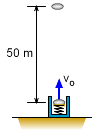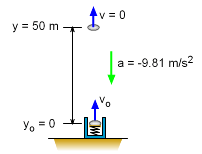Ch 1. Particle General Motion Multimedia Engineering Dynamics Position,Vel & Accel. Accel. varyw/ Time Accel. Constant Rect. Coordinates Norm/Tang. Coordinates Polar Coordinates RelativeMotion
 Chapter - Particle - 1. General Motion 2. Force & Accel. 3. Energy 4. Momentum - Rigid Body - 5. General Motion 6. Force & Accel. 7. Energy 8. Momentum 9. 3-D Motion 10. Vibrations Appendix Basic Math Units Basic Equations Sections Search eBooks Dynamics Fluids Math Mechanics Statics Thermodynamics Author(s): Kurt Gramoll ©Kurt GramollDYNAMICS - EXAMPLEDisc Launching Example A spring loaded machine is able to launch a 2 kg disc 50 meters vertically into the air before it begins to descend. What was the initial velocity? SolutionVelocity will be zero at top Since both the initial velocity and time is unknown, the equation without time should be used,      v2 = vo2 + 2ac(y - yo) Substituting the known values give,      0 = vo2 + 2(-9.81 m/s2)(50 m)      vo = 31.32 m/s

Practice Homework and Test problems now available in the 'Eng Dynamics' mobile app
Includes over 400 problems with complete detailed solutions.
Available now at the Google Play Store and Apple App Store.